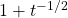# 4.4: Homework- Second Derivatives and Interpreting the Derivative

$$\newcommand{\vecs}{\overset { \rightharpoonup} {\mathbf{#1}} }$$ $$\newcommand{\vecd}{\overset{-\!-\!\rightharpoonup}{\vphantom{a}\smash {#1}}}$$$$\newcommand{\id}{\mathrm{id}}$$ $$\newcommand{\Span}{\mathrm{span}}$$ $$\newcommand{\kernel}{\mathrm{null}\,}$$ $$\newcommand{\range}{\mathrm{range}\,}$$ $$\newcommand{\RealPart}{\mathrm{Re}}$$ $$\newcommand{\ImaginaryPart}{\mathrm{Im}}$$ $$\newcommand{\Argument}{\mathrm{Arg}}$$ $$\newcommand{\norm}{\| #1 \|}$$ $$\newcommand{\inner}{\langle #1, #2 \rangle}$$ $$\newcommand{\Span}{\mathrm{span}}$$ $$\newcommand{\id}{\mathrm{id}}$$ $$\newcommand{\Span}{\mathrm{span}}$$ $$\newcommand{\kernel}{\mathrm{null}\,}$$ $$\newcommand{\range}{\mathrm{range}\,}$$ $$\newcommand{\RealPart}{\mathrm{Re}}$$ $$\newcommand{\ImaginaryPart}{\mathrm{Im}}$$ $$\newcommand{\Argument}{\mathrm{Arg}}$$ $$\newcommand{\norm}{\| #1 \|}$$ $$\newcommand{\inner}{\langle #1, #2 \rangle}$$ $$\newcommand{\Span}{\mathrm{span}}$$$$\newcommand{\AA}{\unicode[.8,0]{x212B}}$$

1. Given each $$f(t)$$, describe in one sentence the meaning of $$f'(t)$$.
1. Let $$f(t)$$ be the distance (in miles) an astronaut is from the surface of the earth as he blasts off towards space. Here $$t$$ is measured in hours.
$$f'(t)$$ is the speed of the astronaut in miles per hour
ans
2. Let $$f(t)$$ be the number of gallons of diesel gasoline in the tank of a truck, with $$t$$ measured in hours.
$$f'(t)$$ is how fast fuel is being burned, in gallons per minute. It could also represent how fast the fuel is being filled up at a gas station.
ans
3. Let $$f(t)$$ be the concentration of NaCl in parts per million within the cytoplasm of a cell. Here, $$t$$ is measured in minutes.
$$f'(t)$$ is the rate the concentration of NaCl is increasing in parts per million per minute.
ans
4. Let $$f(t)$$ be the speed of a runner (in feet per second), and let $$t$$ be measured in seconds.
$$f'(t)$$ is the acceleration of the runner, or the rate at which the runner’s speed is increasing in (feet per second) per second.
ans
5. Let $$f(t)$$ be the rate (in dollars per hour) that you are paid, where $$t$$ is measured in months.
$$f'(t)$$ is like how fast are you getting raises, measured in (dollars per hour) per month.
ans
2. For each of the functions below, compute the derivative twice. That is, compute $$f'(t)$$, then take the derivative of $$f'(t)$$ to find $$f''(t)$$.
1. $$f(t) = 5t^2 - 6t + 2$$
$$10t - 6$$, $$10$$
ans
2. $$f(t) = t^3 + e^t - \sqrt{t}$$.,ans
3. $$f(t) = \frac{1}{t}$$
$$-t^{-2}, 2 t^{-3}$$
ans
4. $$f(t) = \sin(t)$$
$$\cos(t)$$, $$(-\sin(t))$$
ans
5. $$f(t) = (\sqrt{t} + 1)^2$$,ans
3. For each graph, circle any inflection points (if any). Label each region between the inflection points has having either a positive or negative second derivative.ans
4. A river bank is eroding. Let $$f(t)$$ be the metric tonnes of soil and rock material on day $$t$$. Suppose $$f(t) = 0.05t^2 - 3t + 125$$ (this model is valid from $$t = 0$$ to $$t =30$$) .1. What is $$f'(t)$$ measuring? What are the correct units?
How quickly soil and rock are being lost due to erosion in metric tonnes per day.
ans
2. Sketch the derivative.
3. Find the derivative
$$0.1x - 3$$
ans
4. How quickly is material being lost on day $$t = 5$$? How quickly is material being lost on day $$t = 25$$?
$$2.5$$ metric tons per day on day $$5$$, $$0.5$$ metric tons per day on day $$25$$.
ans
5. The stock price for Math Nerds, Inc, over the course of an 8 hour trading day ($$t = 0$$ to $$t = 8$$) is modeled by $$p(t) = -0.3t^3 + 3t^2 - 4t + 17$$ ($$p(t)$$ is measured in dollars).1. What is $$p'(t)$$ measuring? What is $$p''(t)$$ measuring? State the correct units for each.
$$p'(t)$$ is the rate of change of the stock price in dollars per hour. $$p''(t)$$ is how quickly the stock price rate is speeding up or slowing down, measured in dollars per hour$$^2$$
ans
2. Sketch the graph of $$p'(t)$$ and $$p''(t))$$.
3. Compute $$p'(t)$$ and $$p''(t)$$.
$$p'(t) = -0.9t^2 + 6t - 4$$, $$p''(t) = -1.8t + 6$$.
ans
4. How quickly is the stock gaining in price at $$t = 4$$? How quickly is it losing value at $$t = 7$$?
Gaining at 5.6 dollars per hour at $$t = 4$$, but losing at a rate of $$-6.1$$ dollars per hour at $$t = 7$$
ans
5. At $$t = 4$$, the stock price is clearly growing. But is growth speeding up or slowing down? How can you use the formula for $$p(t)$$, $$p'(t)$$, or $$p''(t)$$ to find out?
We can plug $$t = 4$$ into $$p''(t)$$, to get an answer of $$-1.2$$ dollars per hour per hour, which means growth is slowing down.
ans
6. A tank has $$f(t)$$ liters of water at time $$t$$ measured in minutes, where $$f(t) = -2.2t^3 +27t^2- 120t + 500$$ (this model is valid $$t = 0$$ to $$t = 9$$).1. Sketch the graphs of $$f'(t)$$ and $$f''(t)$$.
2. What is $$f'(t)$$ measuring? What is the meaning of $$f''(t)$$? Give the correct units.
Imagine water is leaking out of a hole. $$f'(t)$$ is indirectly measuring the size of that hole, since it is measuring how quickly water is being lost in liters per minute. $$f''(t)$$ would relate to how quickly the hole is opening or closing, thus measuring how fast the rate is changing in liters per minute per minute.
ans

This page titled 4.4: Homework- Second Derivatives and Interpreting the Derivative is shared under a CC BY 4.0 license and was authored, remixed, and/or curated by Tyler Seacrest via source content that was edited to the style and standards of the LibreTexts platform; a detailed edit history is available upon request.